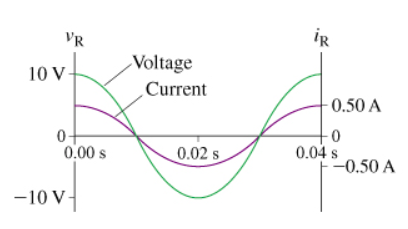# Problem: Draw the resistor's voltage and current phasors at t = 15 ms. The figure (Figure 1) shows voltage and current graphs for a resistor. Draw the vectors with their tails at the origin. The orientation of your vectors will be graded. The exact length of your vectors will not be graded.

###### FREE Expert Solution

Voltage:

$\overline{){\mathbf{V}}{\mathbf{=}}{{\mathbf{V}}}_{{\mathbf{0}}}{\mathbf{c}}{\mathbf{o}}{\mathbf{s}}{\mathbf{\left(}}{\mathbf{\omega }}{\mathbf{t}}{\mathbf{\right)}}}$

Current:

$\overline{){\mathbit{I}}{\mathbf{=}}{{\mathbit{I}}}_{{\mathbf{0}}}{\mathbf{cos}}{\mathbf{\left(}}{\mathbf{\omega t}}{\mathbf{\right)}}}$

Angular frequency:

$\overline{){\mathbf{\omega }}{\mathbf{=}}{\mathbf{2}}{\mathbf{\pi }}{\mathbf{f}}}$

f = 1/T

From the figure, the period T = 0.04s

The period is the time taken to complete one oscillation.

f = 1/0.04 = 25 Hz

ω = (2π)(25) = 157.08 rad/s

88% (151 ratings)###### Problem Details

Draw the resistor's voltage and current phasors at t = 15 ms.

The figure (Figure 1) shows voltage and current graphs for a resistor.Draw the vectors with their tails at the origin. The orientation of your vectors will be graded. The exact length of your vectors will not be graded.